# CLASS 8 MATHS CHAPTER 7-CUBES AND CUBE ROOTS

### Exercise 7.1

Question 1.

Which of the following numbers are not perfect cubes:
(i)216
(ii)128
(iii)1000
(iv)100
(v)46656

Solution :

(i)216
Prime factors of 216 = 2×2×2×3×3×3
Here all factors are in groups of 3’s (in triplets)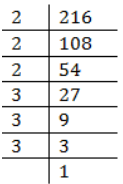Therefore, 216 is a perfect cube number.
(ii) 128
Prime factors of 128
= 2×2×2×2×2×2×2
Here one factor 2 does not appear in a 3’s group.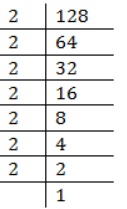Therefore, 128 is not a perfect cube.
(iii) 1000
Prime factors of 1000 = 2×2×2×3×3×3
Here all factors appear in 3’s group.
Therefore, 1000 is a perfect cube.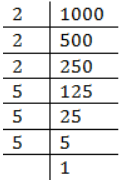(iv) 100
Prime factors of 100 = 2 x 2 x 5 x 5
Here all factors do not appear in 3’s group.
Therefore, 100 is not a perfect cube.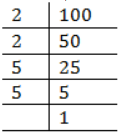(v) 46656
Prime factors of 46656
2x2x2x2x2x2x3x3x3x3x3x3
Here all factors appear in 3’s group.
Therefore, 46656 is a perfect cube.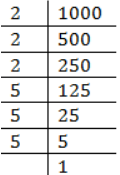Question 2. Find the smallest number by which each of the following numbers must be multiplied to obtain a perfect cube:
(i)243
(ii)256
(iii)72
(iv)675
(v)100

Solution :

(i)243
Prime factors of 243 = 3x3x3x3x3
Here 3 does not appear in 3’s group.
Therefore, 243 must be multiplied by 3 to make it a perfect cube.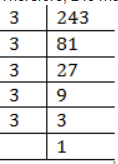(ii) 256
Prime factors of 256
= 2×2×2×2×2×2×2×2
Here one factor 2 is required to make a 3’s group.
Therefore, 256 must be multiplied by 2 to make it a perfect cube.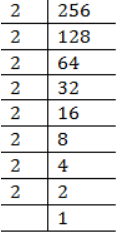(iii) 72
Prime factors of 72 = 2×2×2×3×3
Here 3 does not appear in 3’s group.
Therefore, 72 must be multiplied by 3 to make it a perfect cube.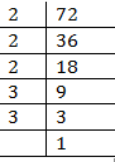(iv) 675
Prime factors of 675 = 3 × 3 × 3 ×  5 × 5
Here factor 5 does not appear in 3’s group.
Therefore 675 must be multiplied by 3 to make it a perfect cube.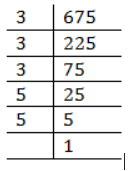(v)  100
Prime factors of 100 =2 × 2 × 5 × 5
Here factor 2 and 5 both do not appear in 3’s group.
Therefore 100 must be multiplied by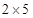= 10 to make it a perfect cube.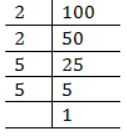Question 3. Find the smallest number by which each of the following numbers must be divided to obtain a perfect cube:
(i)81
(ii)128
(iii)135
(iv)192
(v)704
Solution :

(i)81
Prime factors of 81 = 3 × 3 × 3 × 3
Here one factor 3 is not grouped in triplets.
Therefore 81 must be divided by 3 to make it a perfect cube.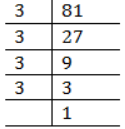(ii) 128
Prime factors of 128 =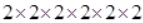Here one factor 2 does not appear in a 3’s group.
Therefore, 128 must be divided by 2 to make it a perfect cube.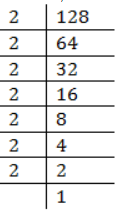(iii) 135
Prime factors of 135 =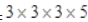Here one factor 5 does not appear in a triplet.
Therefore, 135 must be divided by 5 to make it a perfect cube.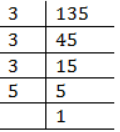(iv) 192
Prime factors of 192 =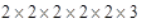Here one factor 3 does not appear in a triplet.
Therefore, 192 must be divided by 3 to make it a perfect cube.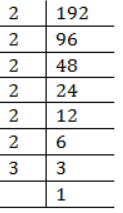(v) 704
Prime factors of 704
=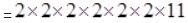Here one factor 11 does not appear in a triplet.
Therefore, 704 must be divided by 11 to make it a perfect cube.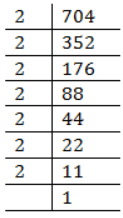Question 4. Parikshit makes a cuboid of plasticine of sides 5 cm, 2 cm, 5 cm. How many such cuboids will he need to form a cube?
Ans. Given numbers =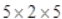Since, Factors of 5 and 2 both are not in group of three.
Therefore, the number must be multiplied by 2 × 5 × 2 = 20 to make it a perfect cube.
Hence he needs 20 cuboids.

### NCERT Solutions for Class 8 Maths Exercise 7.2

Question 1.

Find the cube root of each of the following numbers by prime factorization method:
(i) 64
(ii) 512
(iii) 10648
(iv) 27000
(v) 15625
(vi) 13824
(vii) 110592
(viii) 46656
(ix) 175616
(x) 91125

Solution : (i) 64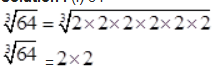= 4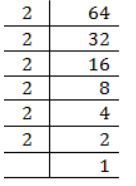(ii) 512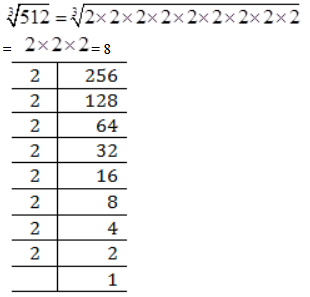(iii) 10648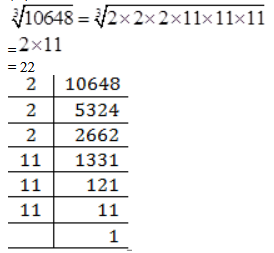(iv) 27000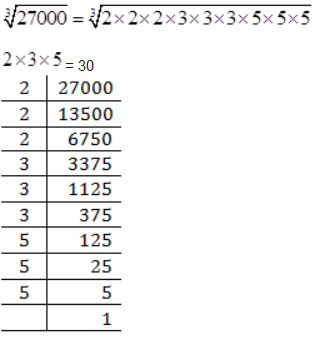(v) 15625

=5 × 5
= 25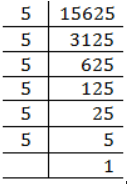(vi) 13824

=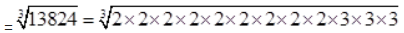= 24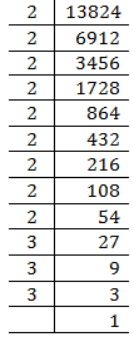(vii) 110592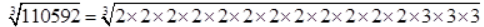2×2×2×2×3
= 48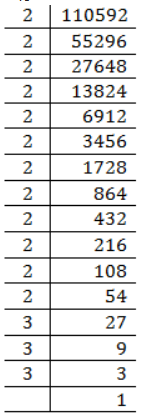(viii) 46656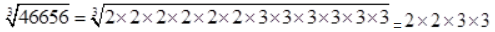= 36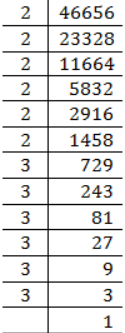(ix) 175616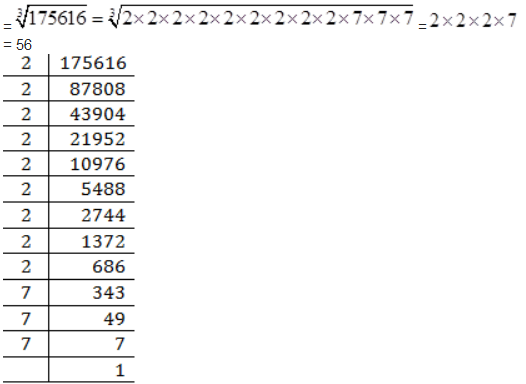= 56

(x) 91125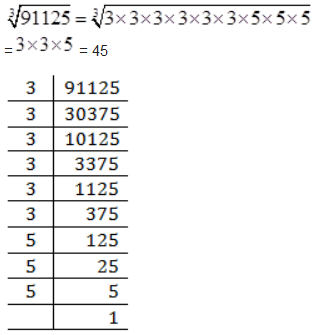Question 2.

State true or false:
(i) Cube of any odd number is even.
(ii) A perfect cube does not end with two zeroes.
(iii) If square of a number ends with 5, then its cube ends with 25.
(iv) There is no perfect cube which ends with 8.
(v) The cube of a two digit number may be a three digit number.
(vi) The cube of a two digit number may have seven or more digits.
(vii) The cube of a single digit number may be a single digit number.

Solution : (i) False
Since,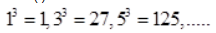are all odd.
(ii) True
Since, a perfect cube ends with three zeroes. e.g.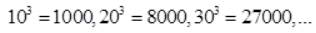so on

(iii) False
Since,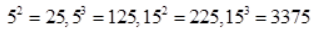(Did not end with 25)

(iv) False
Since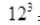= 1728
[Ends with 8]
And  = 10648
[Ends with 8]

(v) False Since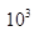= 1000
[Four digit number]
And  11³ = 1331
[Four digit number]
(vi) False Since  99³ = 970299
[Six digit number]

(vii) True
= 1
[Single digit number]
2³ = 8
[Single digit number]

Question 3.

You are told that 1,331 is a perfect cube. Can you guess with factorization what is its cube root? Similarly guess the cube roots of 4913, 12167, 32768.
Solution :

We know that 10³ = 1000 and Possible cube of  11³ = 1331
Since, cube of unit’s digit = 1
Therefore, cube root of 1331 is 11.
4913
We know that  7³ = 343
Next number comes with 7 as unit place  17³ = 4913
Hence, cube root of 4913 is 17.
12167
We know that = 27
Here in cube, ones digit is 7
Now next number with 3 as ones digit
13³ = 2197
And next number with 3 as ones digit
23³ = 12167
Hence cube root of 12167 is 23.
32768
We know that  2³ = 8
Here in cube, ones digit is 8
Now next number with 2 as ones digit
12³ = 1728
And next number with 2 as ones digit
22³ = 10648
And next number with 2 as ones digit
32³ = 32768
Hence cube root of 32768 is 32.

Get 30% off your first purchase!

X
error: Content is protected !!
Scroll to Top